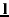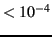### Sources

#### R packages

"ape" (Paradis et al 2004)

"MCMCglmm" (Hadfield, 2010a)

#### Data

Phenotypic count data ("data_pois.txt"), Data frame containing the Poisson count data

Phylogeny ("phylo.nex"), Phylogeny file (NEXUS file)

### Codes

Suppose we have to analyse a dataset alike the one in the OPM section 11.1, but we are now interested in count data without multiple measurement:

 library(ape)
library(MCMCglmm)

 head(data)

##        Zr  N phylo
## 1 0.28918 13  sp_1
## 2 0.02416 40  sp_2
## 3 0.19514 39  sp_3
## 4 0.09831 40  sp_4
## 5 0.13780 66  sp_5
## 6 0.13711 41  sp_6

Because we don't have multiple measurement, we can use the same prior and the same model as in our first example in the OPM section 11.1:
inv.phylo<-inverseA(phylo,nodes="TIPS",scale=TRUE)
prior<-list(G=list(G1=list(V=1,nu=0.02)),R=list(V=1,nu=0.02))
model_pois<-MCMCglmm(phen_pois~cofactor,random=~phylo,
family="poisson",ginverse=list(phylo=inv.phylo\$Ainv),
prior=prior,data=data,nitt=5000000,burnin=1000,thin=500)

Note that we are now using family="poisson", which automatically assumes the canonical logarithmic link function. We can now print the summary of the results:
summary(model_pois)

##
##  Iterations = 1001:4999501
##  Thinning interval  = 500
##  Sample size  = 9998
##
##  DIC: 690.3
##
##  G-structure:  ~animal
##
##        post.mean l-95% CI u-95% CI eff.samp
## animal    0.0403   0.0023    0.109     9998
##
##  R-structure:  ~units
##
##       post.mean l-95% CI u-95% CI eff.samp
## units    0.0421  0.00264   0.0963     9482
##
##  Location effects: phen_pois ~ cofactor
##
##             post.mean l-95% CI u-95% CI eff.samp  pMCMC
## (Intercept)    -2.085   -2.501   -1.693     9667 <1e-04 ***
## cofactor        0.251    0.229    0.273     9998 <1e-04 ***
## ---
## Signif. codes:  0 '***' 0.001 '**' 0.01 '*' 0.05 '.' 0.1 ' ' 1

As we can observe, random effects variances and fixed effects values are low. This is partly due to the assumed logarithmic link function which impose'' low values for the latent trait(see Eqs. (14) and (15) in the main text). However, the fixed effects are significantly different from zero (pMCMC).

Generally, fitting the generalised phylogenetic mixed model using an MCMC algorithm is not much harder than fitting the Gaussian one. However one can encounter several issues. First, the algorithm will be slower for non-Gaussian traits. Second, issues due to auto-correlation might arise, so that one will be forced to run the algorithm for longer. Third, as noted above, the overall expected variances can be much smaller than for Gaussian traits (e.g. for binary and Poisson traits). In this case, issues related to the choice of the prior can arise, especially for small datasets. This is due to the fact that most variance priors (including those available in MCMCglmm) are a bit informative for small variances (for an example of such issues, see de Villemereuil et al, 2013).